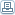# Save A Fortune With A Simple Mortgage

 By: Gerald MasonA simple interest mortgage is a mortgage in which the interest is calculated daily rather than monthly as with a standard mortgage.Contrary to implications of the name, a simple interest mortgage is nothing but simple.However a simple mortgage does have its benefits.First consider a standard mortgage of \$100,000 with a 6% interest rate with interest calculated on a monthly basis.The interest due each month on a standard mortgage is equal to the monthly interest rate multiplied by the balance of the loan. The monthly interest rate is the annual interest rate of 6% divided by the number of months in a year.So in the first month, the interest calculates to .5% multiplied by \$100,000 giving \$500.With a simple interest mortgage, the 6% interest rate is divided by 365, since the interest is calculated daily rather than monthly.In leap years, the annual interest rate is divided by 366.In a typical year, the daily interest rate is .016% (rounded).The interest due for each day is equal to the daily rate multiplied by the balance of the loan.For the first month, it is \$16.44 each day.This \$16.44 accrues each day until the payment is received. When the lender receives a payment for the simple interest mortgage, the payment is first applied to the interest, then to the principle.Since a simple interest mortgage accrues interest on a daily basis, the number of days in the month has an affect on the amount of interest charged.For example, if the first month of the loan has 30 days, the total interest is \$493. However, if the month has 31 days the interest charged is \$510. So, in a 31-day month, the interest on a simple interest mortgage is higher than that of a standard mortgage.If you borrow using a simple interest mortgage, you must be wary of when you send your payments.Since interest on a simple interest mortgage is calculated monthly, there is no grace period as with a standard mortgage. Each day past the due date costs you an additional \$16.44 a day.Since a simple interest mortgage applies payments first to your interest and to your principle second, late payments can cost you more than just the extra amount in interest.If you are more than six days late with your payment during the first month, not a single penny will go toward your principle. Not only that, you could end up with negative amortization, especially if you are more than six days late.Being meticulous with your payments is a must if you have a simple interest mortgage, otherwise, you will find yourself paying more money in interest than necessary.In addition, it could take you longer to pay off your loan.Don't think that just because the lender receives the extra interest when you are late on a simple interest mortgage payment that you are not subject to a late payment charge.Late payment fees still apply. Also consider that "late" to the lender depends on when the payment is posted to your account, not when you wrote the check or when you placed the payment in the mail.